U.S. Department of Transportation
1200 New Jersey Avenue, SE
Washington, DC 20590
202-366-4000

Federal Highway Administration Research and Technology
Coordinating, Developing, and Delivering Highway Transportation InnovationsThis report is an archived publication and may contain dated technical, contact, and link information
 Federal Highway Administration > Publications > Research > Structures > A Laboratory and Field Study of Composite Piles for Bridge Substructures Publication Number: FHWA-HRT-04-043 Date: March 2006

## Chapter 8. ANALYSES OF THE LATERAL LOAD TESTS AT THE ROUTE 351 BRIDGE

### 8.1 INTRODUCTION

An important objective of this research is to determine whether accurate analyses of the lateral load-deflection behavior of composite piles can be performed using the same procedures typically used for prestressed concrete piles and other conventional pile types. If they can, this would remove one of the impediments to using composite piles by verifying that established procedures can be employed for design to resist lateral loads, at least for the type of composite piles studied in this research.

This chapter describes the procedure used for analyzing the lateral load tests of the three test piles at the Route 351 Bridge project. The results of the analyses are compared to the measured responses presented in chapter 6. A brief overview of the laterally loaded pile problem and a description of the methodology used in lateral pile analyses also are presented in this chapter.

### 8.2 GOVERNING DIFFERENTIAL EQUATION FOR THE LATERALLY LOADED PILE PROBLEM

A laterally loaded single pile presents a soil-structure interaction problem. The soil reaction is dependent on the pile movement, and the pile movement is dependent on the soil reaction. The solution must satisfy a nonlinear differential equation as well as equilibrium and compatibility conditions. The solution usually requires several iterations.

Elastic beam relationships that are used commonly in analysis of laterally loaded piles are summarized in table 41. These quantities are obtained from differentiating deflection y with respect to the distance along the pile (x).

Table 41. Relationships commonly

Variable

Formula

Units

Distance along the length of the pile (measured from pile head)

X

[L]

Distance to neutral axis within pile cross section

Z

[L]

Deflection

Y

[L]

Slope or rotation of pile section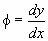[Dimensionless]

Curvature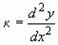Bending moment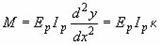[F x L]

Shear force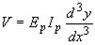[F]

Q

[F]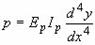[F/L]

Notes: EpIp = flexural stiffness of pile, where Ep = elastic modulus of pile material, and Ip = moment of inertia of pile cross section with respect to the neutral axis.

Figure 179 shows a loaded pile and typical profiles of net soil reaction, deflection, slope, and moment.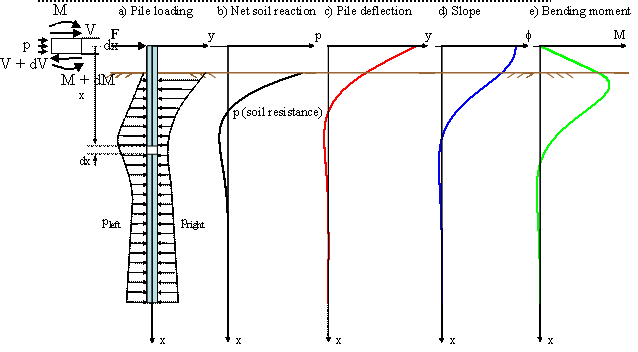Figure 179. Laterally loaded pile problem

The governing differential equation for the problem of a laterally loaded pile was derived by Hetenyi (1946). The differential equation can be obtained by considering moment equilibrium of the infinitesimal element of length (dx) as shown in figure 179.

Neglecting quadratic terms, and differentiating twice with respect to x, we obtain:

The term involving the axial load, Q, can be ignored for the test piles investigated in this research since the vertical load present during testing was mainly from self-weight and can be considered negligible. The magnitude of the bending moment acting at a given section of a pile can be calculated by integrating the normal stresses,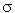(z), acting within the cross section of area, A, as follows:

If we assume that plane sections of the pile remain plane after loading, we can calculate the strains across the pile cross section if we know the rotation of the section,= dy/dx, and the position of the neutral axis. For a given rotation,, we have the following:

where

u(x,z) = is the displacement in the x-direction across the pile cross section

e(z) = strains in the x-direction across the pile cross section

z = distance to the neutral plane

Substituting the expression for(z) from equation 61 into equation 60, we obtain:

If the pile material is linear elastic with a constant Young modulus, Ep, we would have:

Substituting equation 63 into equation 59 and ignoring the axial load term, Q, we obtain:

From consideration of the horizontal force equilibrium of the infinitesimal element of the pile shown in figure 179, we obtain:

Substituting equation 65 into equation 59, we obtain the following governing differential equation, which is commonly used to analyze piles under lateral loads:

The variable, p(x), in equation 66 corresponds to the resultant soil resistance force per unit length of pile that occurs when the unit length of pile is displaced a lateral distance, y, into the soil. A crucial point for solution of the differential equation shown above is adequate representation of the soil reaction, p. If the soil reaction, p, has a linear relationship with lateral pile deflection, y, the equation has a closed-form solution. However, the relationship between the soil reaction (p) and the pile deflection (y) is nonlinear and also varies along the pile depth. In practice, it is common to solve this differential equation (equation 66) using numerical methods such as the finite difference method, and by modeling the soil reaction using nonlinear p-y curves. The analyses presented in this chapter were carried out using this approach. The p-y method used to model the soil reaction is discussed in section 8.3.

### 8.2.1 Assumptions and Limitations of the Governing Differential Equation

Implicit in the derivation of equation 66 is the fact that the pile is made of a homogeneous, isotropic, linear elastic material with the same modulus of elasticity in tension and compression. Hence, the flexural stiffness of the pile, EpIp, is assumed to remain constant during bending. As shown in chapter 6, this assumption is not valid for the piles tested in this research. The nonlinearity of the elastic properties of the piles can be dealt with during the numerical solution of the differential equation by means of successive iterations to account for the nonlinear properties of the structural materials of the pile (Reese and Van Impe 2001).

Another important assumption used in the derivation of equation 66 is that shear strains (or deformations) are small; that is, normals are assumed to remain normal to the neutral axis. This is a common assumption in classical beam theory or Bernoulli-Euler theory. The Mindlin beam theory, on the other hand, assumes normals to the neutral axis remain straight and undeformed, but not necessarily perpendicular to the neutral axis (Holzer, 2001). If we denote withthe angle of rotation of the normal, we have the following for both beam theories:

Bernoulli-Euler theory:= dy/dx

Mindlin theory:= dy/dx +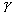If the shear strain,, is zero, the two theories are equal. The Mindlin theory assumes the shear strain is constant over the cross section, that is, independent of z. In reality, the shear strain (or stress) varies over the cross section;varies parabolically over a rectangular cross section made of a uniform material. The effect of shear deformations on beam deflections has been studied by Stippes, et al. (1961). They found that the total tip deflection of a cantilever beam with uniformly distributed load and rectangular cross section is as follows:

where the additional deflection due to shear deformations is given by the second term in the brackets.

The effect of shear deformations increases with increasing E/G ratios and decreasing slenderness ratios (L/D). For the piles tested at the Route 351 Bridge, the slenderness ratio (L/D) is about 15 (considering only the length of the pile where lateral deflections are significant), and the E/2G ratio is estimated to be about 1.3 for the prestessed concrete and FRP piles, and about 5 for the plastic pile. Therefore, the error associated with neglecting shear deformations is estimated to be less than 0.6 percent for the prestressed concrete and FRP piles, and less than 2.2 percent for the plastic pile. However, Han (1997) pointed out the importance of considering shear deformations when studying laterally loaded FRP composite piles. This is believed to be especially important when dealing with thin-walled or hollow FRP beams. Han (1997) reported values of E/2G between 4 and 15 for typical FRP composites materials (with no concrete infill). These E/2G ratios would result in deflection errors between 1.8 percent to 6.7 percent for a slenderness ratio of 15.

### 8.3 METHODOLOGY USED TO ANALYZE THE LATERALLY LOADED TEST PILES

The p-y method is widely used for design of laterally loaded piles. This method replaces the soil reaction with a series of independent nonlinear springs. The p-y curves represent the nonlinear behavior of the soil by relating the soil reaction and pile deflection at points along the pile length. A review of the p-y method is presented in sections 8.3.1 and 8.3.2.

### 8.3.1 P-y Curves

#### 8.3.1.1 Introduction

The prediction of the soil resistance at any point along the pile as a function of pile deflection is perhaps one of the most critical factors in solving the problem of a laterally loaded pile. The distribution of stresses against a cylindrical pile before installation is shown in figure 180(a). At a given depth, the stresses will be uniform and normal to the pile wall (assuming the pile is installed vertically and without inducing bending) (Reese and Van Impe 2001). After the pile is subjected to lateral loading, the pile will deflect and the soil stresses acting on the pile would have a distribution similar to that shown in figure 180(b). It is important to point out that some of the stresses will not be perpendicular to the pile wall due to development of shear stresses at the interface between the pile and the soil. The net soil reaction, p(x), is obtained by integrating the stresses around the pile cross section. The units of p(x) are force per unit length.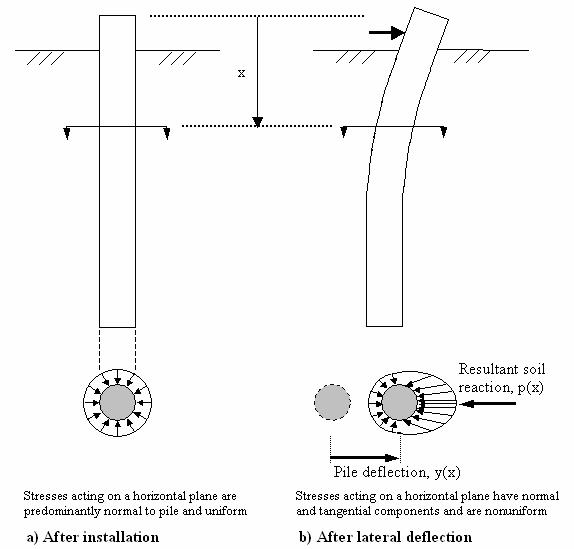Figure 180. Illustration. Distribution of stresses against a pile before and after lateral loading (adapted from Reese and Van Impe 2001).

In general, p-y curves are nonlinear and they are a function of depth, soil type, and pile dimensions and properties. A typical p-y curve is shown in figure 181. Important elements of the p-y curve include the initial slope, Epy-max, and the ultimate soil resistance value, Pult. At any point of the p-y curve the soil reaction, p, is related to the pile deflection, y, through the p-y modulus, Epy (Reese and Van Impe 2001). The p-y modulus is also known as the reaction modulus and it has units of force/length2. Reese and Van Impe (2001) propose using the nomenclature given above instead of the modulus of subgrade reaction, which was originally developed to describe settlement of footings and relates the footing pressure (units of force/length2) to the footing settlement (units of length). These authors also point out that, although the subgrade modulus and Epy are related to the values of the Young modulus of the soil, Es, they are not only a function of the soil, but rather a result of the soil-structure interaction process between the soil and the footing and pile, respectively.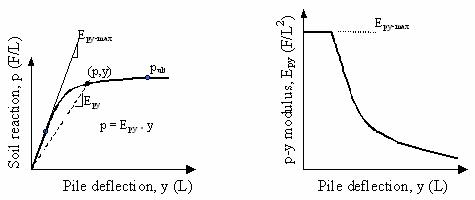Figure 181. Graphs. Typical p-y curve and resulting p-y modulus (Reese and Van Impe 2001).

Ideally p-y curves should be generated from full-scale lateral load tests on instrumented test piles. In the absence of experimentally derived p-y curves, it is possible to use empirical p-y formulations that have been proposed in the literature for different types of soils. Table 42 lists the sources for some of the p-y expressions commonly used in practice.

Table 42. Recommended criteria for p-y curves in different soils (adapted from Reese, et al., 1997).

Soil Type and Condition

Reference

Soft clay below the water table

Matlock (1970)

Stiff clay below the water table

Reese, et al. (1975)

Stiff clay above the water table

Welch and Reese (1972), Reese and Welch (1975)

Sands

Reese, et al. (1974)

Sands

API (1993)

Soils with cohesion and friction

Evans and Duncan (1982)

Weak rock

Reese (1997)

Strong rock

Nyman (1980)

The p-y curves are not defined solely by soil characteristics (Ashour and Norris 2000). In addition to the properties of the soil surrounding the pile, the p-y curves are influenced by several other factors, such as: pile cross-sectional shape and dimensions, interface friction angle between soil and pile, pile bending stiffness, and pile head conditions (Ashour and Norris 2000, Reese and Van Impe 2001). Ashour and Norris (2000) used the strain wedge model to study analytically the influence of some of these factors on p-y curves. They found that for uniform sand deposits, a stiffer pile results in stiffer p-y curves. They also found that if two piles have the same width, but one has a circular cross section and the other has a square cross section, the resulting p-y curves will be different. The square pile in sand showed a soil-pile resistance higher than the circular pile. The findings from Ashour and Norris are based on analytical studies, and to the best of our knowledge no full-scale experiments have been reported to confirm their findings. Reese and Van Impe (2001) also pointed out the influence of the shape of the pile cross section on the soil resistance, p, as illustrated in figure 182.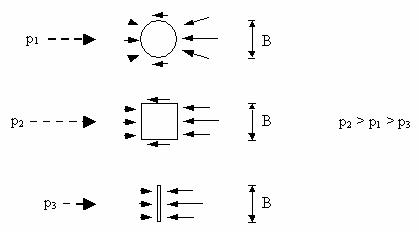Figure 182. Illustration. Schematic showing the influence of shape of cross section of pile on the soil reaction p (adapted from Reese and Van Impe 2001).

The majority of the methods listed in table 42 consider only the influence of the soil properties and the pile width. If other factors such as pile shape and surface texture must be considered, p-y curves should be obtained experimentally based on full-scale tests.

The p-y analyses carried out in this research used published recommendations for p-y curves. The recommendations by Reese, et al. (1974), were used for the sandy soils at the test site. A brief description of these recommendations is provided below.

#### 8.3.1.2 P-y curves for sands based on recommendations by Reese, et al., 1974

The typical shape of a p-y curve for sands, as recommended by Reese, et al., 1974, is shown in figure 183.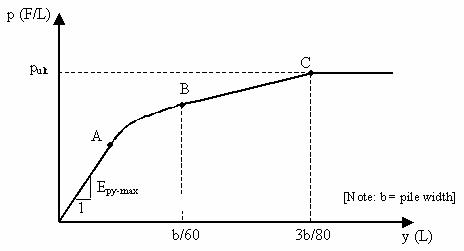Figure 183. Graph. Elements of a characteristic p-y curve for sand based on recommendations by Reese, et al. (1974).

As shown in figure 183, the main elements that define the p-y curves for sands recommended by Reese, et al. (1974), are:

• Initial p-y modulus, Epy-max, that defines the initial portion of the curve up to point A.
• Ultimate soil resistance, pult, which defines the curve at point C and beyond.
• Transition zone between points A and C.

The coordinates of point C are y = 3b/80, and p = pult, where b is the pile width. The transition zone consists of two parts: a parabolic section between points A and B, and a straight line portion between points B and C. The coordinates of point B are defined as:

where A bar subscript s and Bs are coefficients obtained from charts provided by Reese, et al., 1974

The equation of the parabola is obtained knowing that it passes through point B and that it must be tangent to the straight line between points B and C. The coordinates of point A are obtained by finding the intersection point of the initial straight portion, with slope Epy-max, and the parabola.

The LPILE 4.0M program includes this type of p-y curve as a default p-y curve for sands. The p-y curves are generated automatically by the program. The user needs to specify the initial slope of the curve (Epy-max) and the soil properties of the sand (effective unit weight and friction angle) to define the ultimate soil resistance (pult).

Reese, et al. (1974), recommend using a variation of Epy-max that increases linearly with depth, according to:

where

k = a constant giving the variation of Epy-max with depth

x = depth below ground surface

Typical k values for loose and medium-dense sands below the water table are 5.4 and 16.3 MN/m3 (34,403 and 103,847 lbf/ft3), respectively (Reese, et al., 1974).

Before presenting the analyses results, a brief description of the p-y method of analysis is presented in the following section.

### 8.3.2 P-y Method of Analysis

The p-y method of analysis of laterally loaded piles is analogous to the T-Z method described in chapter 7 to analyze the axially loaded piles. In essence, the method consists of dividing the pile into a series of increments of equal length. The governing differential equation (equation 66) is solved using the finite difference technique. The soil is idealized as a series of independent nonlinear springs whose characteristics are represented by the p-y curves described in the previous section. The idealization used in the p-y method is shown in figure 184.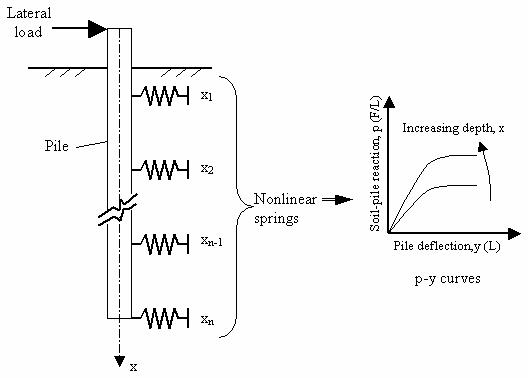Figure 184. Illustration. Schematic showing p-y model used for analysis of laterally loaded piles.

The remainder of this chapter will deal with lateral load analyses carried out for the test piles at the Route 351 project.

### 8.4 NUMERICAL ANALYSES RESULTS

The analyses performed in this research used the p-y method and the computer program LPILE Plus 4.0M (2000). This program employs a finite difference formulation to solve the differential equation presented in the previous section, with nonlinear p-y curves to model the soil. LPILE Plus 4.0M allows computation of the pile response with user-specified nonlinear pile flexural stiffness, EpIp.

The program contains default p-y curves that can be used for different types of soils. As an alternative, the program also allows the user to input p-y curves developed using other formulations. For the analyses carried out in this research, the piles were discretized into 300 elements-the maximum number of increments allowed in the LPILE 4.0M program.

### 8.4.1 General Input Information

#### 8.4.1.1 Pile information

Table 43 summarizes the pile properties used in the analyses. The nonlinear flexural stiffness relationships presented in chapter 6 were used for the three test piles.

Table 43. Properties of test piles.

Property

Prestressed Concrete Pile

FRP Pile

Plastic Pile

Width/diameter (m)

0.610

0.622

0.592

Perimeter (m)

2.44

1.95

1.86

Area (m2)

0.372

0.304

0.275

Length (m)

18.0

18.3

18.3

Initial EpIp (kN-m2)

335,610

186,510

71,705

EpIp versus M

See figure 113(b)

See figure 113(b)

See figure 113(b)

Notes: EpIp = flexural stiffness of pile, M = bending moment. 1 m = 3.28 ft

1 kN-m2 = 2,421 lbf-ft2

#### 8.4.1.2 Upper soil stratigraphy information for p-y analyses

The soil stratigraphy near the top of the pile is the most important when studying laterally loaded piles (Duncan, et al., 1994). Typically, the significant lateral deflections of piles occur within the upper 8 to 10 diameters. The upper soil stratigraphy of the test pile site was found to be somewhat different at the north and south ends of the site.

Figure 185 shows representative in situ test information for the upper 10.0 m (32 ft) of stratigraphy at the northern end of the test pile site. The uppermost layer at the northern end of the test pile site is silty fine sand fill approximately 1.0 m (3.2 ft) thick. The fill is underlain by loose to medium-dense, silty fine sand to a depth of 13.0 m (42.6 ft).

The stratigraphy of the upper 10.0 m (32 ft) at the south end of the test pile site is shown in figure 186. The stratigraphy at the south end consists of 0.5 m (1.6 ft) of silty sand fill, which overlies a medium-stiff sandy, silty, clay layer that extends to about 1.8 m (5.9 ft) depth, which overlies loose to medium-dense silty sand. The extent of the clay layer was determined primarily based on the visual classification of the retrieved split spoon samples from boring SPT-2. The presence of the clay layer was confirmed indirectly via interpretation of the CPT and the flat dilatometer (DMT) test information available for the south end of the test site.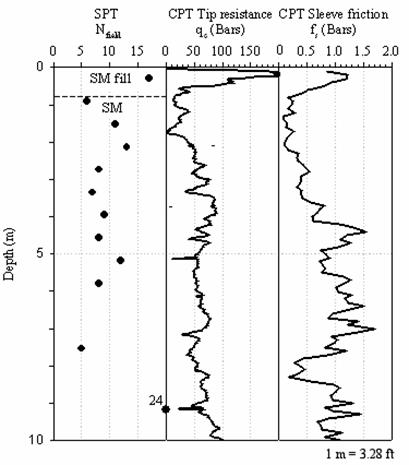Figure 185. Graphs. In situ test data for the upper soils at the northern end of the test pile site.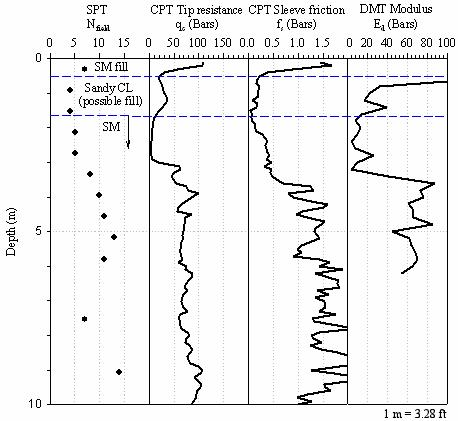Figure 186. Graphs. In situ test data for the upper soils at the southern end of the test pile site.

### 8.4.2 P-y Analyses Results

A series of p-y analyses were carried out using the default p-y curves embedded in the LPILE program for different types of soils. As shown in section 8.4.1.2, the predominant type of soil at the test site consisted of silty sands. The default p-y curves recommended by Reese, et al. (1974), were selected to model these soils.

The uppermost soil layer at the test site consists of manmade fills, with an average thickness of about 1 m and 1.8 m (3.3 ft and 5.9 ft) at the north and south ends of the test site, respectively. Since all three test piles were installed in pits approximately 1.0 m (3.3 ft) deep, the majority of the fill materials were considered to be removed. Therefore, the p-y analyses were carried out using a soil model consisting of predominantly silty sands. However, it is recognized that the clayey fill layer present in the southern end extends about 0.9 m (2.9 ft) beyond the pit bottom of the prestressed concrete pile. This remnant fill was not specifically incorporated in the LPILE model for the test pile in the south side; instead, it was assumed to be part of the underlying silty sand deposit. This approximation was considered reasonable given the sandy and silty nature, and the thickness, of the low plastic clay fill layer. The results of the p-y analyses for the three test piles are summarized in the following sections.

#### 8.4.2.1 Analyses for the prestressed concrete test pile

The prestressed concrete pile is located at the southern end of the test pile site. The surface stratigraphy for this pile is shown in figure 186. The pit excavated for this pile is 0.79 m (2.6 ft) deep. The original ground surface and the point of load application are 1.24 m and 1.34 m (4 ft and 4.4 ft) below the top of the pile, respectively.

The LPILE analyses were carried out using a two-layer model consisting of a layer of loose to medium-dense sand approximately 10 m (32.8 ft) thick, underlain by medium to dense sand. The LPILE model was constructed to take into account the 0.79 m- (2.5 ft-) deep pit excavated before installation of this pile. As mentioned earlier, the silty sands were modeled using the p-y curves recommended for sands by Reese, et al. (1974). The main input information required to define these curves is the initial p-y modulus and the friction angle of the sand. The initial p-y modulus for sands can be adequately modeled as increasing linearly with depth (Reese, et al., 1974). The rate of increase of the p-y modulus was selected based on trial-and-error until a best fit was obtained between the LPILE results and the field measurements. The p-y parameters that provided the best match are summarized in table 44. The initial p-y modulus values used in the analyses are shown in figure 187.

Table 44. Parameters used to define default p-y curves in LPILE for the prestressed concrete pile.

Parameter

Loose Sand

Medium-Dense Sand

Default p-y curve

Reese, et al., 1974

Reese, et al., 1974', Submerged unit weight (kN/m3)

10

11

c, Cohesion (kPa)

0

0, Friction angle (degrees)

33

35

Epy-max, Initial modulus of p-y curve

See figure 187

See figure 187

1 kN/m3 = 6.36 lbf/ft3
1 kPa = 0.145 lbf/inch2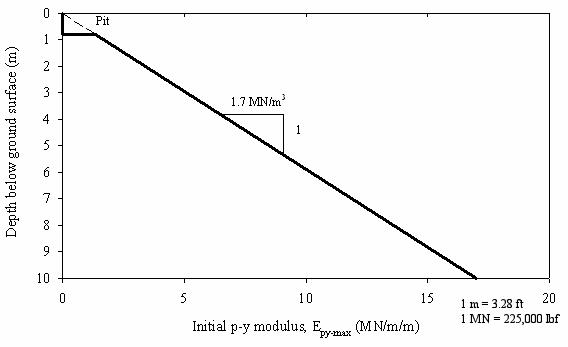Figure 187. Graph. Initial p-y modulus profile used to define p-y default curves for LPILE analyses on the prestressed concrete pile.

The predicted deflected pile shapes are compared to the measured shapes in figures 188 through 190.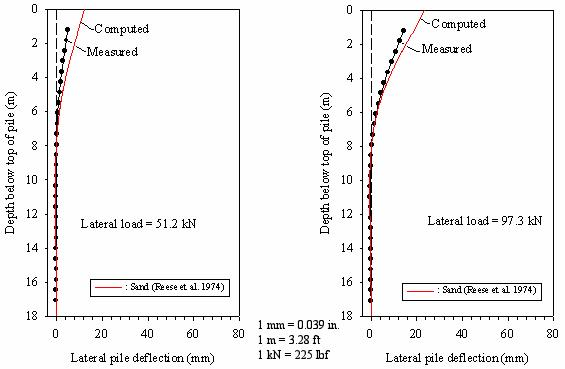Figure 188. Graphs. Predicted versus measured lateral displacement profile for prestressed concrete pile (low lateral loads).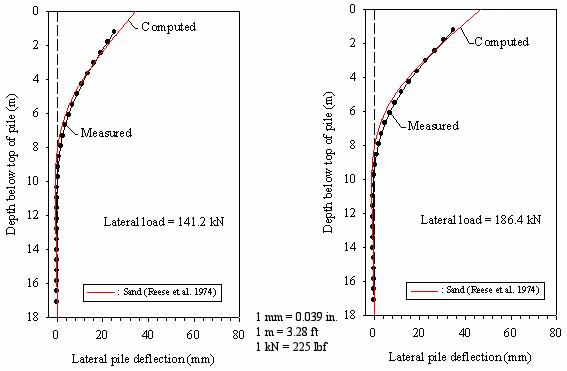Figure 189. Graphs. Predicted versus measured lateral displacement profile for prestressed concrete pile (medium lateral loads).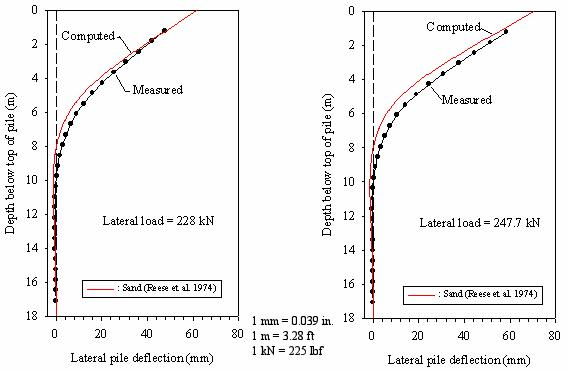Figure 190. Graphs. Predicted versus measured lateral displacement profile for prestressed concrete pile (high lateral loads).

Figures 188-190 show that the deflected shapes of the pile are predicted reasonably well using the p-y modulus (Epy-max) values from figure 187 and the p-y curve equations recommended by Reese, et al., (1974). However, the predictions overestimate the lateral deflections for the two first loads of 51.2 and 97.3 kN (11,520 and 21,893 poundforce), and slightly underpredict the lateral deflections for the higher load levels. This could be related to the characteristics of the Reese, et al., (1974) p-y curves that may be on the "soft" side at low load levels and "stiff" at high load levels. As illustrated in figure 183, the Reese, et al., (1974) curves use a parabola and a straight section to connect the Pult with the initial p-y modulus line. Since there are several possible ways to connect these two states, it is conceivable that other shapes of transition zone will result in different prediction results. A transition zone shape that is stiffer at low load levels and softer at high load levels may be better suited to capture the behavior of the soils encountered at this site. However, the approach was to use the default p-y model that would produce the best level of prediction possible.

Using the LPILE soil model described above, the pile lateral deflections and head rotations at ground surface were calculated. The results are shown in figures 191 and 192.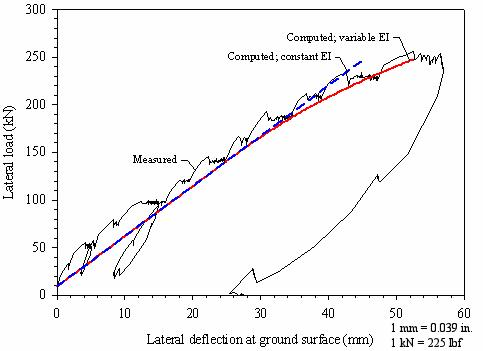Figure 191. Graph. Calculated load-deflection curve for the prestressed concrete pile.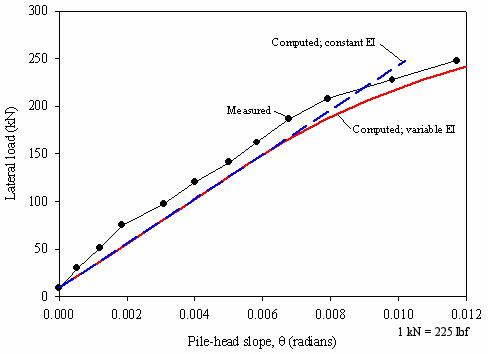Figure 192. Graph. Calculated load-slope curve for the prestressed concrete pile.

The predicted values of lateral deflection and pile head rotation at the ground surface show reasonably good agreement with the field measurements. These figures also show the predicted values if the flexural stiffness of the pile is modeled as being constant, that is, independent of the level of applied moment. The predicted deflections using constant pile flexural stiffness are approximately 7 percent lower than measured at the pile under large lateral loads (> 200 kN (45,000 lbf)), and the agreement is closer at lower lateral loads. This finding is reasonable since the flexural stiffness of the prestressed pile is approximately constant up to a moment of about 400 kN-m (295,028 lbf-ft) (see figure 113(b), chapter 6). Beyond this moment, the flexural stiffness of this pile decreases almost linearly with increasing applied moment. A similar behavior was observed for the head rotations.

#### 8.4.2.2 Analyses for the FRP pile

The FRP pile is located at the northern end of the test pile site. The stratigraphy information for this pile is shown in figure 185. The pit excavated for this pile is 1.06 m (3.5 ft) deep. The original ground surface and the point of load application are 1.09 m (3.6 ft) and 0.79 m (2.6 ft) below the top of the pile, respectively.

For this pile, the LPILE model used in the analyses was constructed using two layers: an upper layer of loose to medium-dense sand, and a lower layer of medium to dense sand. The p-y parameters that provided the best match are summarized in table 45. Both sand layers were modeled using the p-y curves of the type recommended by Reese, et al. (1974).

Table 45. Parameters used to define default p-y curves in LPILE for the FRP pile.

Parameter

Loose Sand

Medium Dense Sand

Default p-y curve

Reese, et al., 1974

Reese, et al., 1974', Submerged unit weight (kN/m3)

10

11

C, Cohesion (kPa)

0

0, Friction angle (degrees)

33

35

Epy-max, Initial modulus of p-y curve

See figure 193

See figure 193

1 kN/m3 = 6.36 lbf/ft3
1 kPa = 0.145 lbf/inch2

The initial p-y modulus values that provided the best fit with the field measurements are shown in figure 193. The predicted deflected pile shapes are compared to the measured shapes in figures 194 through 196.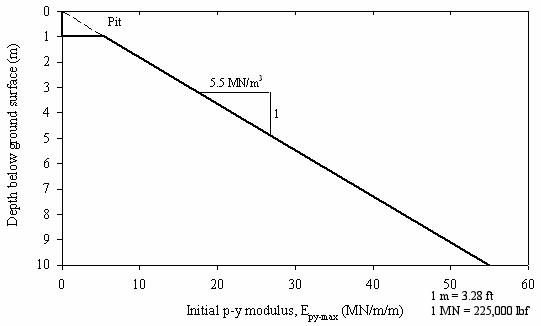Figure 193. Graph. Initial p-y modulus profile used to define default p-y curves for LPILE analyses on the FRP pile.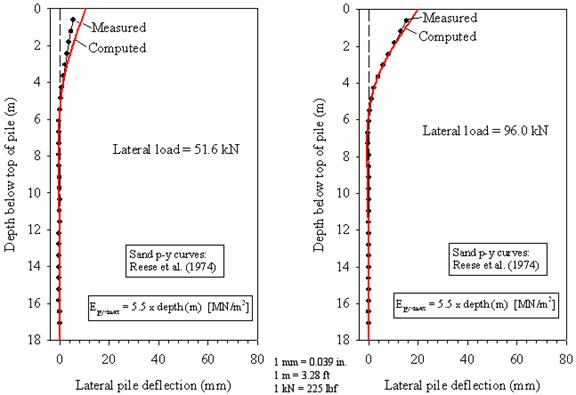Figure 194. Graphs. Predicted versus measured lateral displacement profile for FRP pile (low lateral loads).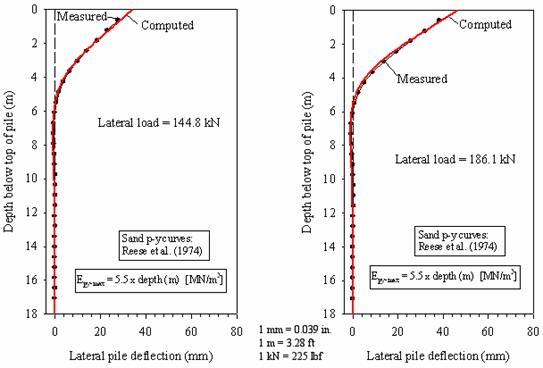Figure 195. Graphs. Predicted versus measured lateral displacement profile for FRP pile (medium lateral loads).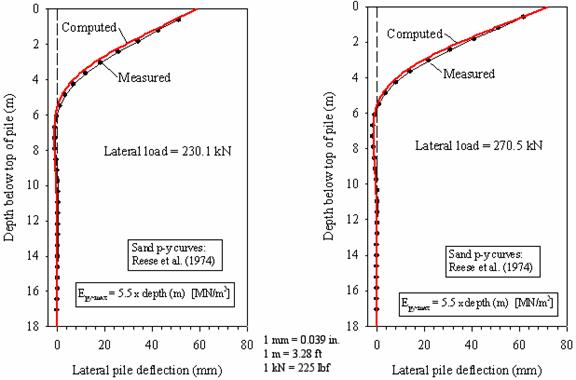Figure 196. Graphs. Predicted versus measured lateral displacement profile for FRP pile (high lateral loads).

These figures show that the pile deflections are predicted reasonably well using the p-y curves recommended by Reese, et al. (1974), for sands and the p-y modulus (Epy-max) values from figure 194. For the first lateral load of 51.6 kN (11,610 lbf), the prediction overestimates the lateral deflections. This could be related to the p-y curve shape characteristics, as for the prestressed concrete pile.

Using the p-y curves given above to model the soil, lateral deflections and pile head rotations were computed for the FRP pile at the ground surface. The results are shown in figures 197 and 198.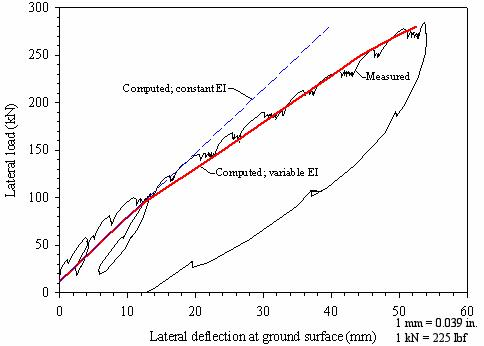Figure 197. Graph. Calculated load-deflection curve for the FRP pile.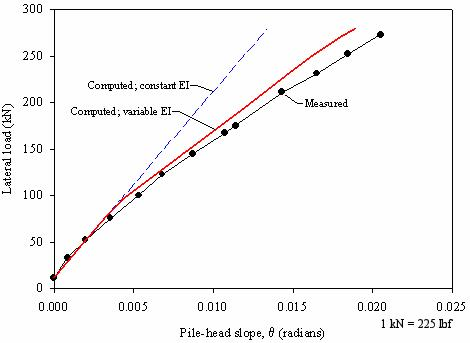Figure 198. Graph. Calculated load-slope curve for the FRP pile.

The predicted values of lateral deflection and pile head rotation at the ground surface show good agreement with the field measurements. These figures also show the calculated values assuming that the flexural stiffness of the pile is constant, that is, independent of the level of applied moment. The predicted deflections using constant pile flexural stiffness are approximately 30 percent lower than measured under large lateral loads (> 150 kN (33,750 lbf)), and the agreement is closer at lower lateral loads. This finding is reasonable since the flexural stiffness of the FRP pile is approximately constant up to a moment of about 200 kN-m (147,514 lbf-ft) (as shown in figure 113(b), chapter 6). Beyond this moment, the flexural stiffness of this pile decreases with increasing applied moment. A similar behavior was observed for the pile head rotations.

#### 8.4.2.3 Analyses for the plastic pile

The plastic pile is located at the center of the test pile site. The extent of the surficial fill at this location was not determined, but is expected to be similar to the conditions found at the south and north ends of the site. The pit excavated for this pile is 0.91 m (3 ft) deep. The original ground surface and the point of load application are 1.02 m and 1.21 m (3.3 ft and 4 ft) below the top of the pile, respectively.

For this pile, like the other piles, the two-layer LPILE model consisted of a layer of loose sand extending 10 m (32.8 ft) deep and an underlying layer of medium to dense sand. The p-y parameters that provided the best match are summarized in table 46. Both sand layers were modeled using the p-y curve types recommended by Reese, et al. (1974).

Table 46. Parameters used to define default p-y curves in LPILE for the plastic pile.

Parameter

Loose Sand

Medium Dense Sand

Default p-y curve

Reese, et al., 1974

Reese, et al., 1974', Submerged unit weight (kN/m3)

10

11

c, Cohesion (kPa)

0

0, Friction angle (degrees)

33

35

Epy-max, Initial modulus of p-y curve

See figure 199

See figure 199

1 kN/m3 = 6.36 lbf/ft3
1 kPa = 0.145 lbf/inch2

The profile of initial p-y modulus with the depth that provided the best match is shown in figure 199. The predicted deflected pile shapes are compared to the measured shapes in figures 200 through 202.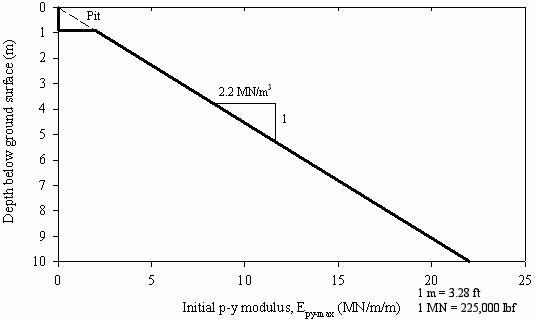Figure 199. Graph. Initial p-y modulus profile used to define default p-y curves for LPILE analyses on the plastic pile.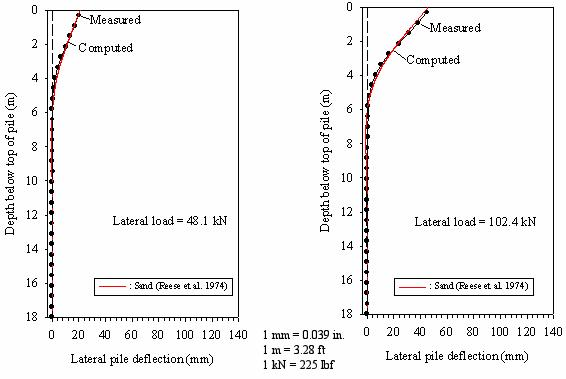Figure 200. Graphs. Predicted versus measured lateral displacement profile for plastic pile (low lateral loads).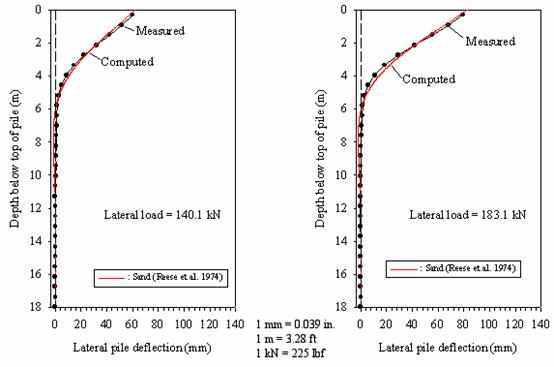Figure 201. Graphs. Predicted versus measured lateral displacement profile for plastic pile (medium lateral loads).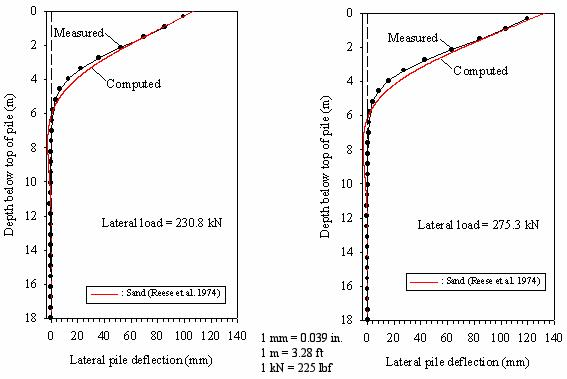Figure 202. Graphs. Predicted versus measured lateral displacement profile for plastic pile (high lateral loads).

Figures 200-202 show that the pile deflections are predicted reasonably well using the p-y curves recommended by Reese, et al. (1974), for sands and the p-y modulus (Epy-max) values from figure 199. The level of agreement between the calculations and measurements was similar for the different load levels, suggesting that the Reese, et al., (1974) p-y curves adequately capture the response measured in the field.

Using the LPILE soil model described above, pile lateral deflections and head rotations at the ground surface were computed. The results are shown in figures 203 and 204.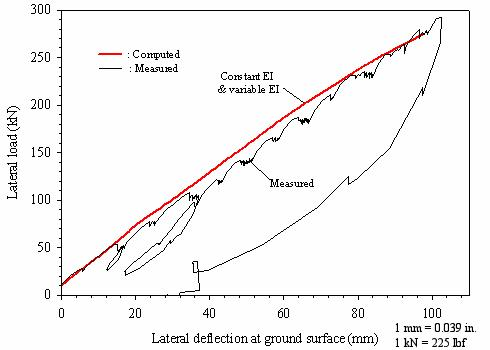Figure 203. Graph. Calculated load-deflection curve for the plastic pile.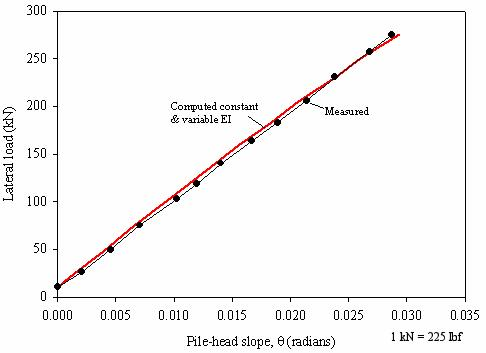Figure 204. Graph. Calculated load-slope curve for the plastic pile.

The calculated values of lateral deflection and pile head rotation at the ground surface show good agreement with the field measurements. For this pile, the predicted values using variable and constant flexural stiffness of the pile are the same. This finding is reasonable because the flexural stiffness of the plastic pile is primarily the result of contributions from the steel rebar cage. Therefore, as shown in figure 113(b) (chapter 6), the flexural stiffness for this pile is approximately constant up to a moment of about 650 kN-m (479,421 lbf-ft). This moment was not exceeded during field load testing.

### 8.4.3 Comparison of the Initial p-y Modulus Curves for the Three Test Piles

The initial p-y modulus for the three test piles was assumed to increase linearly with depth. This assumption was considered reasonable for sand deposits such as those encountered at the test site. The rate of modulus increase with depth was selected to provide the best match between the analytical predictions and the field measurements. Using this approach, we obtained rates of modulus increase with depth of 1.7, 5.5, and 2.2 MN/m3 (10,831, 35,041, and 14,016 lbf/ft3) for the prestressed, FRP, and plastic piles, respectively. These variations are not in agreement with the trend expected based on the results of the axial load tests, for which the average unit shaft capacities were 61.8, 46.9, and 48.9 kPa (8.96, 6.80, and 7.09 (lbf/inch2) for the prestressed concrete pile, the FRP pile, and the plastic pile, respectively.

The differences in the backcalculated rate of increase of the initial p-y modulus with depth can be due to several factors, such as:

• Differences in soil stratigraphy at the location of each test pile. For example, a thin clay layer was encountered at the southern end of the test pile area near the prestressed concrete test pile. This layer was found to extend about 0.9 m (2.9 ft) below the pit at the prestressed concrete pile location.
• Differences in pile properties such as cross-sectional shape, pile width, pile stiffness, surface roughness, and interface friction.

Although the methodology based on double derivation of the bending moment versus depth curves (Reese and Van Impe 2001) is more appropriate for detailed backcalculation of p-y curves, even this method would not have produced p-y curves in accordance with expectations based on the pile characteristics. It seems most likely that the differences in p-y curves are due largely to differences in subsurface conditions.

### 8.5 LIMITATIONS OF P-Y ANALYSES

The p-y method was selected to analyze the lateral load tests carried out at the Route 351 Bridge. This methodology was selected because it is commonly employed in practice. For this research project, it was desirable to verify whether established procedures, such as the p-y method, could be employed to analyze composite piles. Despite the popularity of this method, it has limitations as described below:

• The soil is idealized as a series of independent nonlinear springs represented by p-y curves. Therefore, the continuous nature of the soil is not explicitly modeled.
• The results are very sensitive to the p-y curves used. The selection of adequate p-y curves is the most crucial problem when using this methodology to analyze laterally loaded piles (Reese and Van Impe 2001).
• Selecting appropriate p-y modulus and p-y curves is a difficult task. While the selection of values of the initial p-y modulus (Epy-max) is related to the soil modulus, it is also related to the interaction between the pile and the soil. Reese and Van Impe (2001) point out that p-y curves and modulus are influenced by several pile-related factors, such as:
• Pile type and flexural stiffness.
• Pile geometry.
• Pile cap conditions.
• Pile installation conditions.

### 8.6 SUMMARY

Numerous p-y analyses were carried out to determine the adequacy of this method to analyze laterally loaded composite pile types such as the ones studied in this research project.

A derivation of the governing differential equation for the laterally loaded pile problem was presented, and possible limitations in composite piles analysis were discussed.

The importance of considering shear deformations in lateral pile analyses was discussed. The impact of shear deformations increases with increasing E/G ratios, and decreases with increasing slenderness ratios (L/D). For the test piles assessed in this project, the error associated with neglecting shear deformations is estimated to be less than 2.5 percent.

The importance of including the nonlinearity of the flexural stiffness was discussed and illustrated with the analyses results.

The results of the p-y analyses using published p-y curves that are embedded in the LPILE 4.0M computer program showed reasonably good agreement with the field measurements.

The initial modulus of the p-y curves was found to increase with depth at the highest rate for the FRP pile, at an intermediate rate for the plastic pile, and at the lowest rate for the prestressed concrete pile.

Federal Highway Administration | 1200 New Jersey Avenue, SE | Washington, DC 20590 | 202-366-4000
Turner-Fairbank Highway Research Center | 6300 Georgetown Pike | McLean, VA | 22101